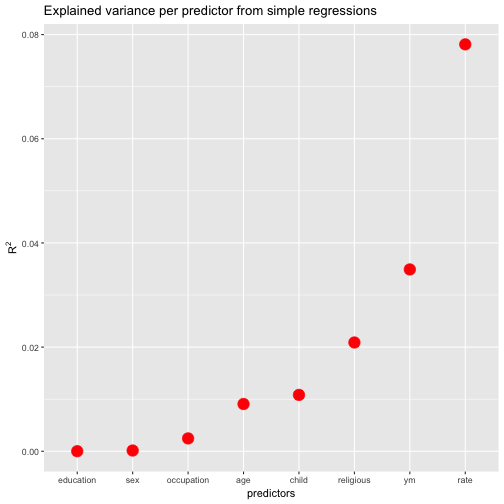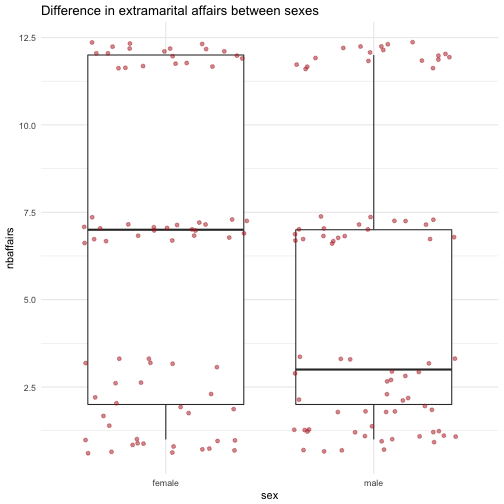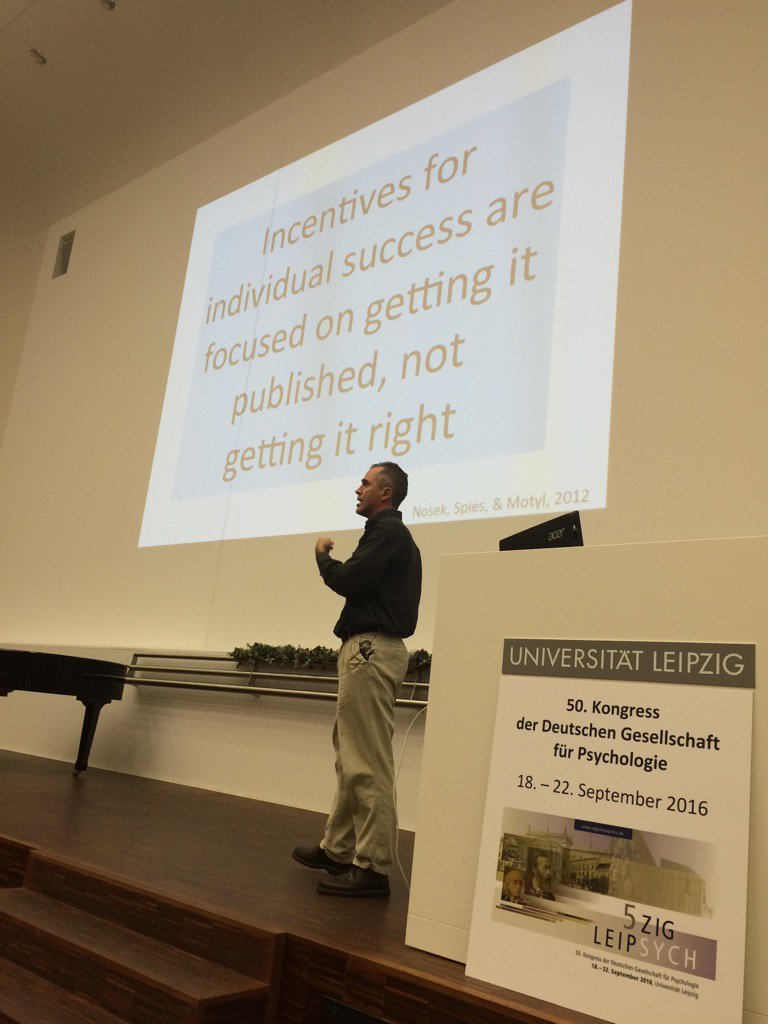# Summary for multiple variables using purrr

A frequent task in data analysis is to get a summary of a bunch of variables. Often, graphical summaries (diagrams) are wanted. However, at times numerical summaries are in order. How to get that in R? That’s the question of the present post.

Of course, there are several ways. One way, using purrr, is the following. I liked it quite a bit that’s why I am showing it here.

First, let’s load some data and some packages we will make use of.

data(Affairs, package = "AER")

library(purrr)
library(dplyr)
library(broom)


Define two helper functions we will need later on:

add_na_col <- function(x){
mutate(x, na = 0)
}

has_n_col <- function(x, n = 6){
return(ncol(x) == n)
}


Set one value to NA for illustration purposes:

Affairs$affairs <- NA # one NA for illustrative purposes  Now comes the show: Affairs %>% select_if(is.numeric) %>% map(~tidy(summary(.x))) %>% # compute tidy summary of each var map_if(., has_n_col, add_na_col) %>% # add na-col if missing do.call(rbind, .) -> Affairs_summary # bind list elements into df Affairs_summary  ## minimum q1 median mean q3 maximum na ## affairs 0.000 0 0 1.458 0.25 12 1 ## age 17.500 27 32 32.490 37.00 57 0 ## yearsmarried 0.125 4 7 8.178 15.00 15 0 ## religiousness 1.000 2 3 3.116 4.00 5 0 ## education 9.000 14 16 16.170 18.00 20 0 ## occupation 1.000 3 5 4.195 6.00 7 0 ## rating 1.000 3 4 3.932 5.00 5 0  What we did was: 1. Get the Affairs data, and select the numeric columns 2. Map the summary function to each column, and tidy up each column. We will get a list of tidy summaries. 3. If a list element has 6 elements (or columns, because we want to end up with a data frame), then we know there is no NA-column. In this case, add_na_col, else not. That’s what the map_if bit does. 4. Lastly, bind the list elements row wise. To that end, give a bag of summary-elements to rbind by help of do.call. Instead of purr::map, a more familiar approach would have been this: Affairs %>% dplyr::select_if(is.numeric) %>% lapply(., function(x) tidy(summary(x))) # compute tidy summary of each var  ##$affairs
##   minimum q1 median  mean   q3 maximum na
## 1       0  0      0 1.458 0.25      12  1
##
## $age ## minimum q1 median mean q3 maximum ## 1 17.5 27 32 32.49 37 57 ## ##$yearsmarried
##   minimum q1 median  mean q3 maximum
## 1   0.125  4      7 8.178 15      15
##
## $religiousness ## minimum q1 median mean q3 maximum ## 1 1 2 3 3.116 4 5 ## ##$education
##   minimum q1 median  mean q3 maximum
## 1       9 14     16 16.17 18      20
##
## $occupation ## minimum q1 median mean q3 maximum ## 1 1 3 5 4.195 6 7 ## ##$rating
##   minimum q1 median  mean q3 maximum
## 1       1  3      4 3.932  5       5


And, finally, a quite nice formatting tool for html tables is DT:datatable (output not shown):

library(DT)
datatable(Affairs_summary)


Although this approach may not work in each environment, particularly not with knitr (as far as I know of).

That’s why an alternative html table approach is used:

library(htmlTable)

htmlTable(Affairs_summary)

minimum q1 median mean q3 maximum na
affairs 0 0 0 1.458 0.25 12 1
age 17.5 27 32 32.49 37 57 0
yearsmarried 0.125 4 7 8.178 15 15 0
religiousness 1 2 3 3.116 4 5 0
education 9 14 16 16.17 18 20 0
occupation 1 3 5 4.195 6 7 0
rating 1 3 4 3.932 5 5 0

# EDIT: Running multiple simple regressions with purrr

EDIT based on comments/ suggeestions from @JonoCarroll Disqus profile and @tjmahr twitter profile. See below (last step; look for “EDIT”).

Thanks for the input! :thumbsup:

reading time: 10 min.

Hadley Wickham’s purrr has given a new look at handling data structures to the typical R user (some reasoning suggests that average users doesn’t exist, but that’s a different story).

I just tried the following with purrr: - Meditate about the running a simple regression, FWIW - Take a dataframe with candidate predictors and an outcome - Throw one predictor at a time into the regression, where the outcome variable remains the same (i.,e multiple simple regressions (one predictor) where the predictor is changed at each run but the outcome remains the same) - tidy up the resulting $R^2$ in some nice format

I found that purrr does the job nicely, and it’s quite instructive to see purrrat work, I think. That’s why I wrote it up in this short post:

library(purrr)
library(ggplot2)
library(dplyr)
library(broom)
library(knitr)  # for kable
data(Fair, package = "Ecdat") # extramarital affairs dataset
glimpse(Fair)

## Observations: 601
## Variables: 9
## $sex <fctr> male, female, female, male, male, female, female, ... ##$ age        <dbl> 37, 27, 32, 57, 22, 32, 22, 57, 32, 22, 37, 27, 47,...
## $ym <dbl> 10.00, 4.00, 15.00, 15.00, 0.75, 1.50, 0.75, 15.00,... ##$ child      <fctr> no, no, yes, yes, no, no, no, yes, yes, no, yes, y...
## $religious <int> 3, 4, 1, 5, 2, 2, 2, 2, 4, 4, 2, 4, 5, 2, 4, 1, 2, ... ##$ education  <dbl> 18, 14, 12, 18, 17, 17, 12, 14, 16, 14, 20, 18, 17,...
## $occupation <int> 7, 6, 1, 6, 6, 5, 1, 4, 1, 4, 7, 6, 6, 5, 5, 5, 4, ... ##$ rate       <int> 4, 4, 4, 5, 3, 5, 3, 4, 2, 5, 2, 4, 4, 4, 4, 5, 3, ...
## $nbaffairs <dbl> 0, 0, 0, 0, 0, 0, 0, 0, 0, 0, 0, 0, 0, 0, 0, 0, 0, ...  Fair %>% dplyr::select(-nbaffairs) %>% # exclude outcome, leave only predictors map(~lm(Fair$nbaffairs ~ .x, data = Fair)) %>%
map(summary) %>%
map_dbl("r.squared") %>%
tidy %>%
dplyr::arrange(desc(x)) %>%
rename(r.squared = x) -> r2s

kable(r2s)

names r.squared
rate 0.0781272
ym 0.0349098
religious 0.0208806
child 0.0108181
age 0.0090701
occupation 0.0024613
sex 0.0001377
education 0.0000059

Ok, that appears to be the list of the $R^2$ for each simple (one-predictor) regression we have run.

Let’s do a quick sense check with the standard way:

lm1 <- lm(nbaffairs ~ rate, data = Fair)

summary(lm1)

##
## Call:
## lm(formula = nbaffairs ~ rate, data = Fair)
##
## Residuals:
##     Min      1Q  Median      3Q     Max
## -3.9063 -1.3989 -0.5631 -0.5631 11.4369
##
## Coefficients:
##             Estimate Std. Error t value Pr(>|t|)
## (Intercept)   4.7421     0.4790   9.900   <2e-16 ***
## rate         -0.8358     0.1173  -7.125    3e-12 ***
## ---
## Signif. codes:  0 '***' 0.001 '**' 0.01 '*' 0.05 '.' 0.1 ' ' 1
##
## Residual standard error: 3.17 on 599 degrees of freedom
## Multiple R-squared:  0.07813,	Adjusted R-squared:  0.07659
## F-statistic: 50.76 on 1 and 599 DF,  p-value: 3.002e-12

summary(lm1)$r.squared  ##  0.07812718  summary(lm1)$coefficients[2, 4]  #p.value

##  3.002385e-12


Seems to work. To get details of the object summary(lm1), use str(summary(lm1)).

How many did we run? Just the number of columns minus one (the outcome variable).

ncol(Fair)-1

##  8


FWIW, let’s plot the resulting values (and sort the predictors by descending values).

ggplot(r2s, aes(x = reorder(names, r.squared), y = r.squared)) +
geom_point(size = 5, color = "red") +
ylab(expression(R^{2})) +
xlab("predictors") +
ggtitle("Explained variance per predictor from simple regressions")Wait, one more thing. Suppose we are not only interested in $R^2$, but in the p-values (OMG). How to get both values from purrr?.

EDIT (the following part has changed)

Thanks for comments from @JonoCarroll Disqus profile and @tjmahr twitter profile, the last step - extracting the p-values - is now changed, and I think improved.

Fair %>%
dplyr::select(-nbaffairs) %>%  # exclude outcome, leave only predictors
map(~lm(Fair$nbaffairs ~ .x, data = Fair)) %>% map(summary) %>% map(broom::tidy) %>% map_df("p.value") %>% round(3) %>% mutate(variable = c("intercept", "predictor")) -> ps  library(htmlTable) htmlTable(ps)  sex age ym child religious education occupation rate variable 1 0 0.465 0.019 0 0 0.1 0.002 0 intercept 2 0.774 0.02 0 0.011 0 0.952 0.225 0 predictor So what I did above basically is: • Run a linear model on each predictor • Get a summary of each model • Tidy (with broom) each summary • Get the sublist (column) p.value from each list (model), and save the result as a data frame To get a whole bunch of relevant statistics, you can use glance: Fair %>% dplyr::select(-nbaffairs) %>% # exclude outcome, leave only predictors map( ~lm(Fair$nbaffairs ~ .x, data = Fair)) %>%
map(summary) %>%
map_df(glance) %>%
round(3)

##   r.squared adj.r.squared sigma statistic p.value df
## 1     0.000        -0.002 3.301     0.083   0.774  2
## 2     0.009         0.007 3.287     5.483   0.020  2
## 3     0.035         0.033 3.243    21.667   0.000  2
## 4     0.011         0.009 3.284     6.551   0.011  2
## 5     0.021         0.019 3.267    12.774   0.000  2
## 6     0.000        -0.002 3.302     0.004   0.952  2
## 7     0.002         0.001 3.297     1.478   0.225  2
## 8     0.078         0.077 3.170    50.764   0.000  2


Thanks for comments from @JonoCarroll Disqus profile and @tjmahr twitter profile, the last step - extracting the p-values - is now changed, and I think improved.

# Running multiple simple regressions with purrr

Hadley Wickham’s purrr has given a new look at handling data structures to the typical R user (some reasoning suggests that average users don’t exist, but that’s a different story).

I just tried the following with purrr:

• Meditate about the running a simple regression, FWIW
• Take a dataframe with candidate predictors and an outcome
• Throw one predictor at a time into the regression, where the outcome variable remains the same (i.,e multiple simple regressions (one predictor) where the predictor is changed at each run but the outcome remains the same)
• tidy up the resulting $R^2$ in some nice format.

I found that purrr does the job nicely, and it’s quite instructive, I think. That’s why I wrote it up in this short post:

library(purrr)
library(ggplot2)
library(dplyr)
library(broom)
library(knitr)  # for kable
data(Fair, package = "Ecdat") # extramarital affairs dataset
glimpse(Fair)

## Observations: 601
## Variables: 9
## $sex <fctr> male, female, female, male, male, female, female, ... ##$ age        <dbl> 37, 27, 32, 57, 22, 32, 22, 57, 32, 22, 37, 27, 47,...
## $ym <dbl> 10.00, 4.00, 15.00, 15.00, 0.75, 1.50, 0.75, 15.00,... ##$ child      <fctr> no, no, yes, yes, no, no, no, yes, yes, no, yes, y...
## $religious <int> 3, 4, 1, 5, 2, 2, 2, 2, 4, 4, 2, 4, 5, 2, 4, 1, 2, ... ##$ education  <dbl> 18, 14, 12, 18, 17, 17, 12, 14, 16, 14, 20, 18, 17,...
## $occupation <int> 7, 6, 1, 6, 6, 5, 1, 4, 1, 4, 7, 6, 6, 5, 5, 5, 4, ... ##$ rate       <int> 4, 4, 4, 5, 3, 5, 3, 4, 2, 5, 2, 4, 4, 4, 4, 5, 3, ...
## $nbaffairs <dbl> 0, 0, 0, 0, 0, 0, 0, 0, 0, 0, 0, 0, 0, 0, 0, 0, 0, ...  Fair %>% dplyr::select(-nbaffairs) %>% # exclude outcome, leave only predictors map(~lm(Fair$nbaffairs ~ .x, data = Fair)) %>%
map(summary) %>%
map_dbl("r.squared") %>%
tidy %>%
dplyr::arrange(desc(x)) %>%
rename(r.squared = x) -> r2s

kable(r2s)

names r.squared
rate 0.0781272
ym 0.0349098
religious 0.0208806
child 0.0108181
age 0.0090701
occupation 0.0024613
sex 0.0001377
education 0.0000059

Ok, that appears to be the list of the $R^{2}$ for each simple (one-predictor) regression we have run.

Let’s do a quick sense check with the standard way:

lm1 <- lm(nbaffairs ~ rate, data = Fair)

summary(lm1)

##
## Call:
## lm(formula = nbaffairs ~ rate, data = Fair)
##
## Residuals:
##     Min      1Q  Median      3Q     Max
## -3.9063 -1.3989 -0.5631 -0.5631 11.4369
##
## Coefficients:
##             Estimate Std. Error t value Pr(>|t|)
## (Intercept)   4.7421     0.4790   9.900   <2e-16 ***
## rate         -0.8358     0.1173  -7.125    3e-12 ***
## ---
## Signif. codes:  0 '***' 0.001 '**' 0.01 '*' 0.05 '.' 0.1 ' ' 1
##
## Residual standard error: 3.17 on 599 degrees of freedom
## Multiple R-squared:  0.07813,	Adjusted R-squared:  0.07659
## F-statistic: 50.76 on 1 and 599 DF,  p-value: 3.002e-12

summary(lm1)$r.squared  ##  0.07812718  summary(lm1)$coefficients[2, 4]  #p.value

##  3.002385e-12


Seems to work. To get details of the object summary(lm1), use str(summary(lm1)).

How many did we run? Just the number of columns minus one (the outcome variable).

ncol(Fair)-1

##  8


FWIW, let’s plot the resulting values (and sort the predictors by descending values).

ggplot(r2s, aes(x = reorder(names, r.squared), y = r.squared)) +
geom_point(size = 5, color = "red") +
ylab(expression(R^{2})) +
xlab("predictors") +
ggtitle("Explained variance per predictor from simple regressions")Wait, one more thing. Suppose we are not only interested in $R^{2}$, but in the p-values (OMG). How to get both values from purrr?.

library(magrittr)

Fair %>%
dplyr::select(-nbaffairs) %>%  # exclude outcome, leave only predictors
map(~lm(Fair$nbaffairs ~ .x, data = Fair)) %>% map(summary) %>% map(c("coefficients")) %>% map_dbl(8) %>% # 8th element is the p-value tidy %>% dplyr::arrange(desc(x)) %>% rename(p.value = x) -> ps kable(ps)  names p.value education 0.9524501 sex 0.7740138 occupation 0.2245709 age 0.0195320 child 0.0107275 religious 0.0003797 ym 0.0000040 rate 0.0000000 Extracting the p-value by map_dbl(8) is surely far from perfect. Any ideas how to better get the value out of this numeric 2*4 matrix? Thoughts are welcome! # Code example for plotting boxplots instead of mean bars On a recent psychology conference I had the impression that psychologists keep preferring to show mean values, but appear less interested in more detailled plots such as the boxplot. Plots like the boxplot are richer in information, but not more difficult to perceive. For those who would like to have an easy starter on how to visualize more informative plots (more than mean bars), here is a suggestion: # install.pacakges("Ecdat") library(Ecdat) # dataset on extramarital affairs data(Fair) str(Fair)  ## 'data.frame': 601 obs. of 9 variables: ##$ sex       : Factor w/ 2 levels "female","male": 2 1 1 2 2 1 1 2 1 2 ...
##  $age : num 37 27 32 57 22 32 22 57 32 22 ... ##$ ym        : num  10 4 15 15 0.75 1.5 0.75 15 15 1.5 ...
##  $child : Factor w/ 2 levels "no","yes": 1 1 2 2 1 1 1 2 2 1 ... ##$ religious : int  3 4 1 5 2 2 2 2 4 4 ...
##  $education : num 18 14 12 18 17 17 12 14 16 14 ... ##$ occupation: int  7 6 1 6 6 5 1 4 1 4 ...
##  $rate : int 4 4 4 5 3 5 3 4 2 5 ... ##$ nbaffairs : num  0 0 0 0 0 0 0 0 0 0 ...

library(ggplot2)
library(dplyr)

Fair %>%
filter(nbaffairs != 0) %>%
ggplot(aes(x = sex, y = nbaffairs)) +
ggtitle("Difference in extramarital affairs between sexes") +
geom_boxplot() +
geom_jitter(alpha = .5, color = "firebrick") +
theme_minimal()As can be seen, the distribution information reveals some more insight than bare means: There appear to be three distinct groups of “side lookers” (persons having extramarital relations).

This would not come out if looking at means only:

Fair %>%
na.omit() %>%
group_by(sex) %>%
summarise(mean_affair_by_sex = mean(nbaffairs)) %>%
ggplot(aes(x= sex, y = mean_affair_by_sex)) +
geom_bar(stat = "identity") +
ggtitle("Mean value difference in extramarital affairs between sexes")# How to promote open science? Some practical recommendations

I just attended the biannual conference of the German society of psychology (DPGs) in Leipzig; open science was a central, albeit not undisputed topic; a lot of interesting related twitter discussion.image source: Felix Schönbrodt

Interestingly, a strong voice of German scientiests uttered their concerns about being scooped if/when sharing their data (during the official meeting of the society). This being said (sad), the German research foundation (DFG) has updated its guidelines now stressing (more strongly) that publicly funded projects should share their data, with the rationale that the data do not belong to the individual scientiest but to the public, as the public funded it (I find that convincing). Finally, Brian Nosek had a key note talk, where he vividly argued in favor of open science; I found the talk very inspiring.

Anyway, I do not want to discuss the “why” and “if” but rather the “how” in this post, giving some recommendations. Or more precisely, what I personally do at the moment for living up to open science. The following list is by no means innovative, impressive, it just presents my current thinking and doing:

• Share you code, e.g., on Github
• Spread the word (blog your thoughts), Github and Jekyll provide a cool tool
• Sign the (or one of the) research transparency initiatives for reviewers, and live up to it
• Publish your data, e.g., on Open Science Framework (OSF)
• Publish a data paper, e.g., here, and get a citable paper out of in it, including a doi
• Checkout whether your pay-walled journal accepts preprint/postprint open access (many do!) (BTW: Here’s a blog post explaining differences between preprints and postprints, and what your rights are)
• Put your preprint/postprint on a repository, eg., OSF or SocArXiv
• If you are editor or faculty head: promote open science in your environment
• Preregister your study, eg. on OSF
• Learn more about good data (management/analysis) practices, e.g, on OSF
• Connect on social media, e.g, Twitter hashtag #openscience
• Consider becoming an ambassador of open science at COS

Peronally, I put my code on Github, and my data and preprints to OSF (and other stuff, such as presentations, too). And I blog and twitter on that (among other topics).

The list above is surely not exhaustive, nor it is definitely mandatory to submit to each item. I am to still learning and getting more into it.

I think we should consider that it may well be that our research results are mostly false, see Paul Meehls seminal paper(s), and of course the results of the Reproducibilty Project Psychology, among many others sources.

Hopefully, we will not drift astray in emotional discussions on “research parasites”, “methodological terrorists”, or “cyberbullies”, but focus on what is constructive for our field. One could argue that the only question that counts is “How do I contribute?”.